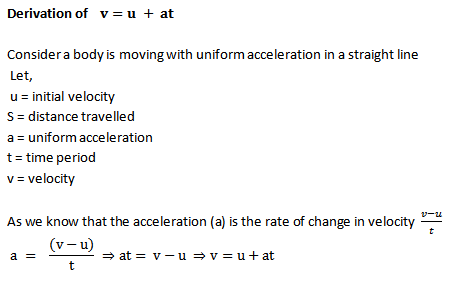# Derivation of equations of motion

Derivation of equations of motion

Derivation of The Equations of MotionDerivation of S = ut + ½ at2Derivation of v2 - u2 = 2as